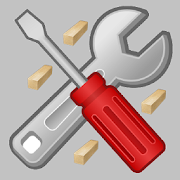# Handyman CalculatorEveryone
22,178
Complete construction calculator with simple inputs. The app for construction and DIY! Reduce Waste, Save Time and Money! Well tested by more than a million contractors and home owners! Top 100 productivity app. Give it a try!

Calculate materials, track invoices, time spent, manage to-do list and save calculations to notepad.

Listed in "This Old House" magazine "The TOH Top 100: Best New Home Products 2013" and Bob Vila newsletter.

The best contractor app for construction!

Time Tracking tool
To-do list
CutList calculator
Feet and inches calculator
Square footage calculator
Construction calculator
Measurement calculator
Contractor calculator
Carpenter calculator
Woodworking calculator
Asphalt calculator
Construction material calculators
Plywood Calculator
Framing calculator
Building materials calculators
Construction project calculators
Carpenters calculator
Flooring calculator
Brick calculator
Bid calculator
Project calculator
Plywood calculator
Job estimate calculator
Feet inches calculator
Imperial calculator
Angle calculator
Lumber calculator
Woodworker Calculators
Concrete Calculator
Siding calculator
Electrical calculators
Metric units arithmetic
Feet,inches TO Metre,cm,mm Converter
Tape measure calculator

Fraction Calculator
Fraction Simplification
Cubic Footage Calculator
Lumber,Asphalt calculator

Length, Area and Weight Converter.
Temperature Converter
Density Converter
Board Foot Calculator
Board feet and Linear feet Converter
Lumber Weight Calculator
Deck Baluster Calculator
Deck Board Calculator
Spacing Calculator
Wall Framing Calculator
Firewood Calculator and BTU Chart
Lumber Log Volume calculator
Concrete, Brick, Block, Mortar and Sand Calculations
Excavation Calculator
Asphalt Volume calculator
Aggregate Calculator
Drywall calculator
Crown Molding Calculator
Carpet Calculator
Laminate Flooring Calculator
Wall Paper Calculator
Tile Calculator
Paint Calculator
Stairs Calculator
Arc Length Calculator
Air Conditioning and Heater Calculator
Roof Calculator
Roof Pitch Angle Chart
Rafter Length Calc
Rebar Weight Chart
Mulch and Topsoil Calculator
Patio Pavers Calculator
Brick Calculator
Plants Calculator.
Wood Fence Calculator
Grass Seed Calculator
Sod Calculator
Blown Insulation R value Calculator
Golden Ratio(Golden mean) Calculator
Speed, Distance and Time Calculator
Rain water Collection Calculator
Loan Calculator
Speed and Velocity Unit Converter
Water Flow Rate Calculator.
Tank Capacity Calculator
Ohm's Law Calculator
Resistor Color Code Calculator
Resistance Conversion
Power Calculator
Amps to Watts Calculator
Capacitance, Frequency, Inductance and Resistance Calculator
Voltage Drop and divider Calculator
Wire Size Calculator
Power Wash Calculator
Sine Bar Calculator
Wrench Conversion Chart
Decimal and Fraction Converter
Diagonal calculator
Slope Calculator
Right Triangle Calculator
Rectangle Calculator
Square Calculator
Circle Calculator
Polygon Calculator
Fraction to Percentage Calculator
Angle Converter
Power Converter
Energy Converter
Torque Converter
Force Units Converter
Liquid Units Converter
Pressure Converter
Magnetic Flux Converter
Volume FlowRate Units Converter
Degrees, Minutes, Seconds and Decimal Degrees Conversion
BMI Calculator
Miles Per Gallon (MPG) and Kilometers Per Litres Calculator
Gas and Oil mixture Ratio Calculator
Discount Calculator
Date Duration Calculator
Tip Calculator
Graphing Calculator
PCB Trace Width Calculator
World Clock
Shipment Density Calculator
Currency Converter
Oil Drilling Calculators
Refrigerant Pressure Temperature Chart

Mowing Calculator
Electric Charge Converter
Fuel Consumption Converter
Enthalpy Conversion
Led Resistor Calculator including Series and Parallel
Frequency, Wavelength and Energy Converter
Milling Machine calcs
Mat border calculator for picture frames
Tap and Drill Chart
Woodturners Segment Calculator
Lumens to Watts Calculator
Metal detector
Collapse

Review Policy
4.5
22,178 total
5
4
3
2
1

## What's New

Fixed app icon
Collapse

Updated
May 22, 2018
Size
6.8M
Installs
1,000,000+
Current Version
2.4.6
Requires Android
4.0 and up
Content Rating
Everyone
Permissions
Offered By
Handyman Calculator
Developer
P.O. Box 3604, Olathe, KS, 66063